Home About Us Practice Test GMAT eBooks Math Books GMAT Classes Forums B SchoolsGMAT Frequently Asked Questions GMAT CAT Test Details / Signup GMAT Syllabus, Testing Subject areas GMAT Math Syllabus, Sample QuestionseBook on Number Theory and Inequalities eBook on Permutation & Combination eBook on Geometry Doubts, discussions in GMAT Math Share your GMAT Test experience 4GMAT's Achievers' List TestimonialsFree GMAT Questions Answers Free GMAT Math Test GMAT Blogs GMAT Quant Blogs GMAT FAQs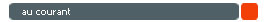Top US B Schools R1/R2 deadlines Featured B School Rearranging numbers - Permutation Counting number of 1s and 0s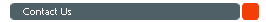Chennai : 95000 48484 Mumbai : 80970 48484 Bangalore : 91419 48484 eMail : info@4gmat.comYou are here: 4GMAT Home » GMAT FAQ » GMAT Verbal Section » GMAT Integrated Reasoning (IR)

# GMAT Integrated Reasoning (IR) : What is the IR section all about?

The Integrated Reasoning or the IR section was introduced in the GMAT since June 2012. This section replaced the GMAT AWA - Issue essay. In the earlier version of the GMAT, a test taker had to write two essays in the first 1 hour. Now, the test taker has to write one essay - Analysis of an Argument for 30 minutes and then the new IR section for another 30 minutes.

# Quick Facts about the IR Section

1. Duration of this section: 30 minutes

2. Number of questions: 12 questions

3. Type of questions
1. Graphic Interpretation
2. Two Part Analysis
3. Table Analysis
4. Multi Source Reasoning

4. Scoring Range and Interval: 1 to 8 in intervals of 1

# a. Sample Graphic Interpretation Question

In this question type, the test taker is expected to interpret a graph or graphical image and select the option from a drop-down list to make the answer statements accurate.

### Question

The graph given below provides data about the total cost in billions of dollars and the profit in millions of dollars of a trading firm from 2004 to 2009. The axis on the left represents the total cost and the axis on the right represents the profit.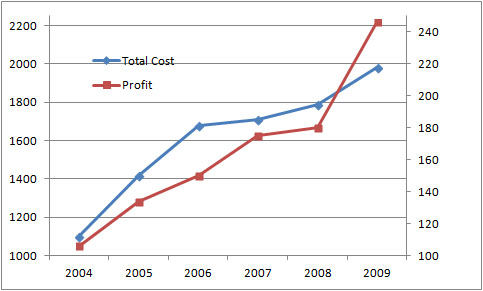Fill in the blanks in following statements suitably based on the information given in the graph using the options in the drop down menu

1. The rate of growth of total cost is     the rate of growth of profits from 2004 to 2009.

2. The percent change in total cost from 2004 to 2005 is approximately     the percent change in profit from 2005 to 2006.

# b. Sample Two Part Analysis Question

In this question type, the test taker is expected to solve a problem with a two-part solution. Possible answers will be presented in a table with a column for each part.

### Question

A merchant marks her wares up by p% above her cost C and then offers a discount on the marked price. In terms of the variables p and C, select the expression that represents the % discount offered if the merchant makes a profit of 40% while selling at the discounted price and select the expression that represents the selling price if she offers a discount of 20% to the marked price.

 % discount % Selling Price 0.8C + 0.08pC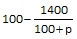0.8pC+0.08C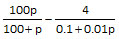0.8C+0.008pC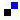Add to del.icio.us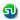Stumble Itdigg this